•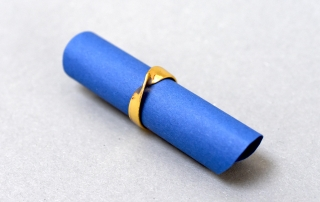Moebius Strip ring
Gallery

Moebius Strip ring

The Moebius Strip is a mathematical classic, and even for rings it has been used for many decades at least. Our version of the Moebius Strip ring, however, is special. Its shape is defined by a mathematical formula!

•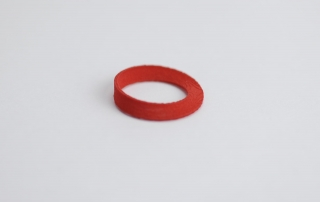Moebius Strip pendant
Gallery

Moebius Strip pendant

The Moebius Strip is a mathematical classic, and even for pendants it has been used for many decades at least. Our version of the Moebius Strip pendant, however, is special. Its shape is defined by a mathematical formula!

•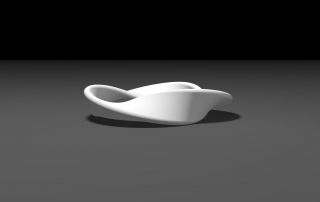Moebius Strip pendant
Gallery

Moebius Strip pendant

The Moebius Strip is a mathematical classic, and even for rings it has been used for many decades at least. Our version of the Moebius Strip pendant, however, is special. Its shape is defined by a mathematical formula!

•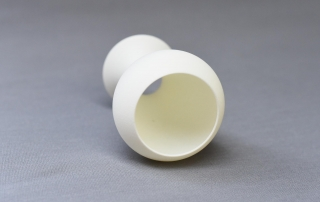A math vase of degree 3 without bottom
Gallery

A math vase of degree 3 without bottom

A math vase of degree 3 without bottom. It has been created by rotating a graph of a polynomial of degree 3 about an axis.

•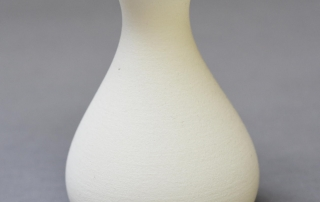A math vase of degree 3
Gallery

A math vase of degree 3

A math vase of degree 3. It has been creating by rotating a graph of a polynomial of degree 3 about an axis.

•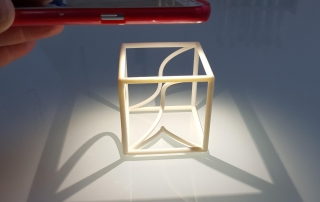Space curve in a cube with projections (1b)
Gallery

Space curve in a cube with projections (1b)

This space curve in a cube with projections (1b) is a math classic. Use a torch to compare the projections of the space curve (in the center) on a plane with the 3d-printed ones.

Space curve in a cube without projections (1a)

This space curve in a cube is a math classic. To view its projections on a plane, just take a torch (or your cell phone lamp).

•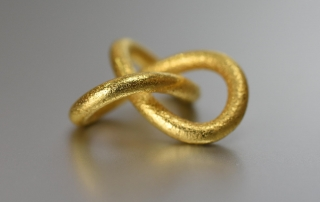A trefoil knot pendant
Gallery

A trefoil knot pendant

A trefoil knot is the simplest non-trivial mathematical knot. It has been known for thousands of years.

•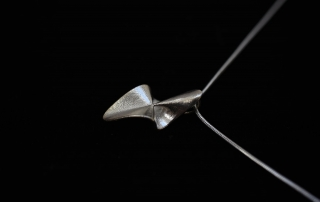Cubic surface KM 42 pendant
Gallery

Cubic surface KM 42 pendant

Cubic surfaces are a math model classic from the 19th century. We provide one of our favourite examples (cubic surface KM 42) in the form of a pendant.

•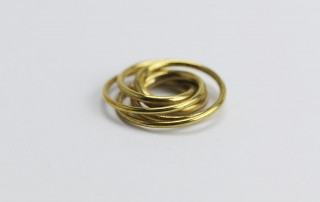Speed Curve pendant
Gallery

Speed Curve pendant

At first sight, our speed curve pendant might seem to consist of some arbitrary wire. But this is not true at all. Almost every detail is defined by mathematical formulas.